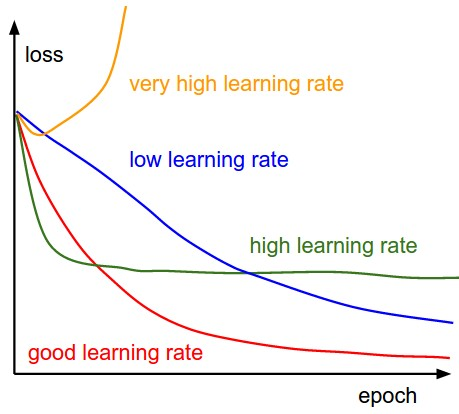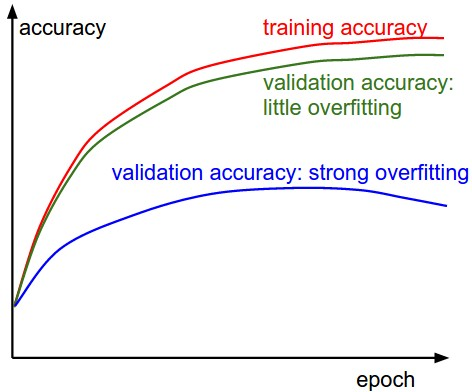/How to train your Neural Network## How to train your Neural Network

The value of a neural network lies in its hyper-tuning.

## General intuition

The VA(validation accuracy) of your NN(Neural network) is always going to be less than TA(train accuracy). So if the maximum TA it gets is 60%, don’t expect the VA to be more than 60. Hence, your first concern should be to push TA as high as possible and then use regularisation in the form of dropout to push the VA. We achieve this by tuning our hyperparameters.

First overfit and then regularise~

## Hyperparameters

Hyperparameter optimization or tuning is the problem of choosing a set of optimal hyperparameters for a learning algorithm. The same kind of machine learning model could require different constraint, weights or learning rates to generalize different data patterns. These measures are called hyperparameters, and have to be tuned so that the model can best solve the machine learning problem.

Neural Network hyperparameters:

• Activation function
• Weight initialization
• Number of layers
• Number of units in a layer
• Learning rate
• Dropouts at different layers
• Optimiser for loss function
• Train-Test data split value
• Number of epochs

### Activation functions

To understand the activation functions, their pros and cons, I highly suggest this article. It clearly explains the backprop algorithm which is at the heart of NN. After this, understand why we should choose ReLu(Rectified Linear Unit) over other activation functions by this post. I have gone through several posts but the clarity and sincerity of these 2 posts remain unmatched. If you are going to apply for Deep learning(DL) roles, it is very likely that interviewer will ask questions on backprop and ReLu.Although there are several pros and cons to using the ReLUs:

• (Pros) Compared to sigmoid/tanh neurons that involve expensive operations (exponentials, etc.), the ReLU can be implemented by simply thresholding a matrix of activations at zero. Meanwhile, ReLUs does not suffer from saturating.
• (Pros) It was found to greatly accelerate the convergence of stochastic gradient descent compared to the sigmoid/tanh functions. It is argued that this is due to its linear, non-saturating form.
• (Cons) Unfortunately, ReLU units can be fragile during training and can “die”. For example, a large gradient flowing through a ReLU neuron could cause the weights to update in such a way that the neuron will never activate on any datapoint again. If this happens, then the gradient flowing through the unit will forever be zero from that point on. That is, the ReLU units can irreversibly die during training since they can get knocked off the data manifold. For example, you may find that as much as 40% of your network can be “dead” (i.e., neurons that never activate across the entire training dataset) if the learning rate is set too high. With a proper setting of the learning rate this is less frequently an issue.

Leaky ReLuLeaky ReLUs are one attempt to fix the “dying ReLU” problem. Instead of the function being zero when x<0, a leaky ReLU will instead have a small negative slope (of 0.01, or so). That is, the function computes f(x)=alpha x if x<0 and f(x)=x if xgeq 0, where alpha is a small constant. Some people report success with this form of activation function, but the results are not always consistent.

Parametric ReLU

In PReLU, the slopes of negative part are learned from data rather than pre-defined.

Nowadays, a broader class of activation functions, namely the rectified unit family, were proposed.From these tables, we can find the performance of ReLU is not the best for all the three data sets. For Leaky ReLU, a larger slope alpha will achieve better accuracy rates. PReLU is easy to overfit on small data sets (its training error is the smallest, while testing error is not satisfactory), but still outperforms ReLU. In conclusion, three types of ReLU variants all consistently outperform the original ReLU in these three data sets. And PReLU and RReLU seem better choices.

TLDR: Use Paramteric ReLu with α ~ 0.1

### Weight initialization#### Weight initialisation criterias:

• All weights should not be same – Same weights will contribute in same proportion to the output and get rectified in same proportion by backprop leading to a viscous cycle of same weights in all passes
• Weight values should not be high – The sigmoid activation function fires ~1 for weight value >7. This means that it is agnostic to weight value more than 7. But the bigger problem is that slope of sigmoid remains almost constant for weight value >7 which kills the weight update in backprop i.e. the neural network training get stuck• Weight value should depend on the nodes in the layer connected by the weights i.e the weight is associated between 2 layers and the number of neurons in the incoming layer and target layer will affect the weight values

All these criterias are taken care by Xavier-Glorot initialisation scheme and a comparison of all the methods is shown here.

### Number of layers

The number of layers mostly depends on the complexity you are dealing with. Deeper is considered better than flatter. Go on adding layers until the accuracy increases.

### Number of units in a layer

There is no optimal size for this. Once you fix the architecture, increase the units in fully connected and filters in CNN layers until the accuracy goes on increasing.

### Learning rate

If you are training with SGD or Nesterov, it’s very important to choose an appropriate learning rate at various points of epoch. You can skip all this mess by choosing Adam optimiser which chooses learning rate automatically.### Dropouts at different layers

After you confirm that you have successfully overfitted the model try to regularise it by dropout. Add dropouts after fully connected layers. Use less dropout values in initial layers (0.2-0.3) and more(upto 0.5) in last layers. Don’t use dropouts on convolutional layers because they will spoil the idea of CNN. You can use them on fully connected and recurrent layers(LSTM, GRU)

### Optimisers

The loss value has to be reduced by optimising the loss function which is done by the optimisers in Tensorflow. Have a look at this video to understand each of them in detail.

### Number of epochsThere is no perfect number for it. Just see the plot of train and test accuracy. The moment test accuracy starts falling and train accuracy keeps increasing is the point where you should stop. That’s the best accuracy you are going to get out of your current network.

### Tips to train effectively• Train accuracy not increasing:
• Increase the number of units in layers
• Increase the number of layers
• Increase the number of epochs if you don’t see TA falling after a certain epoch. The point at which TA starts falling is the point after which the network has started over-fitting
• Increase the training data by changing the train/test split value. Don’t give more than 90% data to train
• Shuffle data and then split into train/test if you haven’t. If test contains classes that train has never seen, the network will predict incorrectly
• Augment data – Used a lot in case of images. (Let me know if you have a nice way to augment text data using wordnet and word vectors)
• Check for imbalanced classes – Decide the minimum data-points per class required to train the NN and then put all the classes with less data-points into ‘other’ category. Don’t remove these classes because when they will be encountered in real time, we would like it to be classified as other
• Train accuracy fluctuates up and down a lot
• Try reducing your learning rate if you are using SGD or Nestorov
• Try using Adam optimiser which takes care of choosing learning rate at different states of training
• Train accuracy high but validation accuracy is low
• Add dropouts after fully connected layers if you haven’t
• Try increasing dropout values

## Optimization algorithms

Since a lot of iterations of these parameters are required to effectively the train the model, we might as well just automate the process. There are several methods for this as discussed below.

Grid search

The traditional way of performing hyperparameter optimization has been grid search, or a parameter sweep, which is simply an exhaustive searching through a manually specified subset of the hyperparameter space of a learning algorithm. A grid search algorithm must be guided by some performance metric, typically measured by cross-validation on the training set or evaluation on a held-out validation set.

Since the parameter space of a machine learner may include real-valued or unbounded value spaces for certain parameters, manually set bounds and discretization may be necessary before applying grid search.

For example, a typical soft-margin SVM classifier equipped with an RBF kernel has at least two hyperparameters that need to be tuned for good performance on unseen data: a regularization constant C and a kernel hyperparameter γ. Both parameters are continuous, so to perform grid search, one selects a finite set of “reasonable” values for each, sayGrid search then trains an SVM with each pair (C, γ) in the Cartesian product of these two sets and evaluates their performance on a held-out validation set (or by internal cross-validation on the training set, in which case multiple SVMs are trained per pair). Finally, the grid search algorithm outputs the hyperparameter configuration that achieved the highest score in the validation procedure.

Bayesian optimization

Bayesian optimization is a methodology for the global optimization of noisy black-box functions. Applied to hyperparameter optimization, Bayesian optimization consists of developing a statistical model of the function from hyperparameter values to the objective evaluated on a validation set. Intuitively, the methodology assumes that there is some smooth but noisy function that acts as a mapping from hyperparameters to the objective. In Bayesian optimization, one aims to gather observations in such a manner as to evaluate the machine learning model the least number of times while revealing as much information as possible about this function and, in particular, the location of the optimum. Bayesian optimization relies on assuming a very general prior over functions which when combined with observed

In Bayesian optimization, one aims to gather observations in such a manner as to evaluate the machine learning model the least number of times while revealing as much information as possible about this function and, in particular, the location of the optimum. The methodology proceeds by iteratively picking hyperparameters to observe (experiments to run) in a manner that trades off exploration (hyperparameters for which the outcome is most uncertain) and exploitation (hyperparameters which are expected to have a good outcome). In practice, Bayesian optimization has been shown to obtain better results in fewer experiments than grid search and random search, due to the ability to reason about the quality of experiments before they are run.

Random search

Since grid searching is an exhaustive and therefore potentially expensive method, several alternatives have been proposed. In particular, a randomized search that simply samples parameter settings a fixed number of times has been found to be more effective in high-dimensional spaces than exhaustive search. This is because oftentimes, it turns out some hyperparameters do not significantly affect the loss.